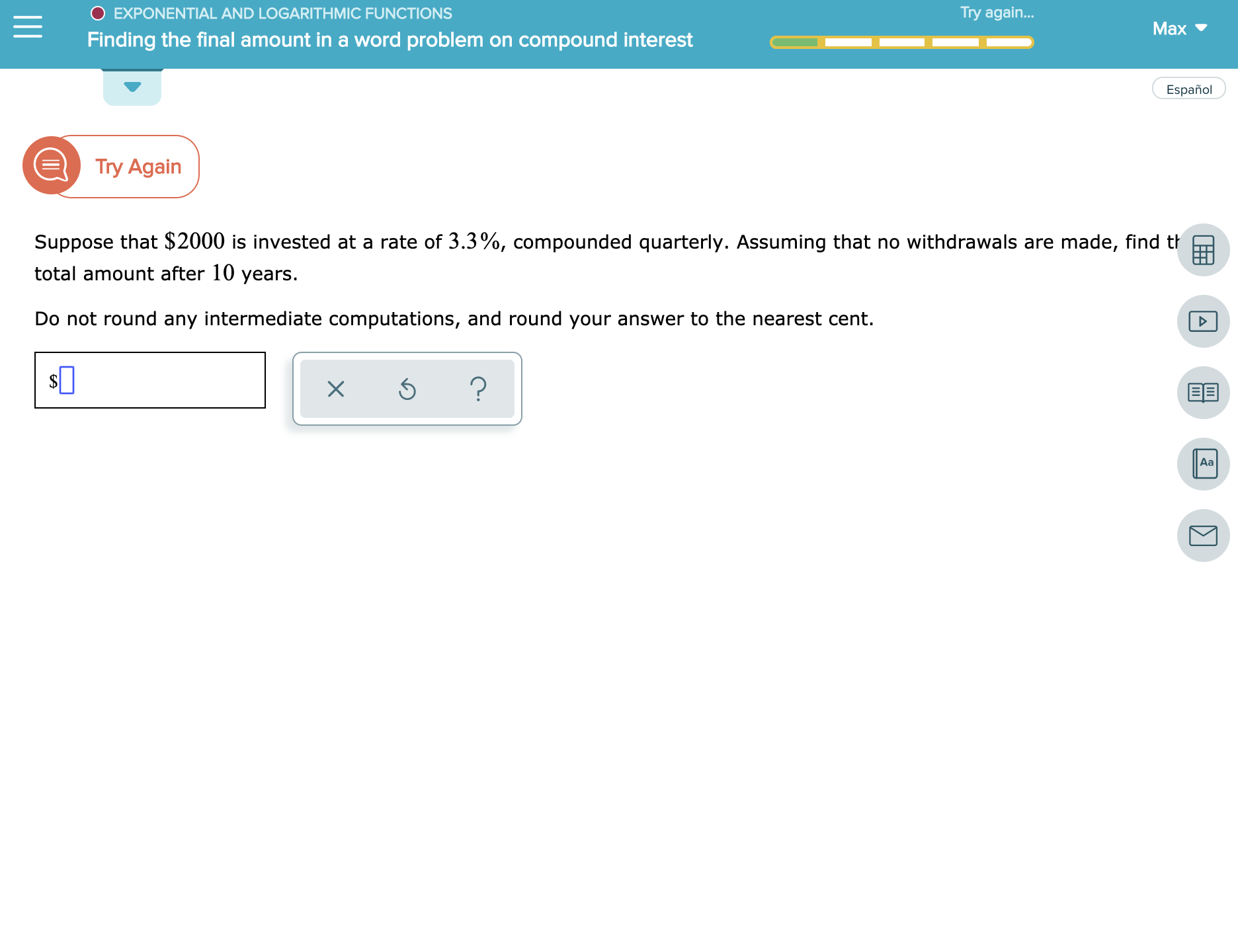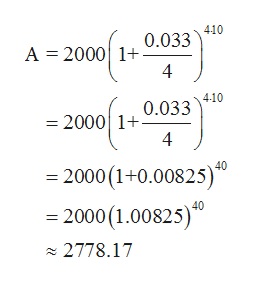# O EXPONENTIAL AND LOGARITHMIC FUNCTIONSTry agai...MaxFinding the final amount in a word problem on compounde interestEspañolETry AgainSuppose that \$2000 is invested at a rate of 3.3%, compounded quarterly. Assuming that no withdrawals are made, find thtotal amount after 10 years.Do not round any intermediate computations, and round your answer to the nearest cent.?XAa

Question
3 views

See attachmenthelp_outlineImage TranscriptioncloseO EXPONENTIAL AND LOGARITHMIC FUNCTIONS Try agai... Max Finding the final amount in a word problem on compounde interest Español ETry Again Suppose that \$2000 is invested at a rate of 3.3%, compounded quarterly. Assuming that no withdrawals are made, find th total amount after 10 years. Do not round any intermediate computations, and round your answer to the nearest cent. ? X Aa fullscreen
check_circle

Step 1

To find the amount present in bank after time t years we use the below formula:
where,
P = Principal or amount of money deposited
n = number of times compounded per year
r = annual interest rate
t = times in years
A = total amount after t years.

Step 2

Given:
Amount of money deposited, P = \$2000/-
Interest Rate, r = 3.3%
number of times compounded per year, N = 4
Time, t = 10 years

Step 3

Plugging these values in the a...help_outlineImage Transcriptionclose0.033 410 A 2000 1 4 4-10 =2000 10.033 4 =2000 (1+0.00825) 40 2000 (1.00825) 2778.17 fullscreen

### Want to see the full answer?

See Solution

#### Want to see this answer and more?

Solutions are written by subject experts who are available 24/7. Questions are typically answered within 1 hour.*

See Solution
*Response times may vary by subject and question.
Tagged in

### Other